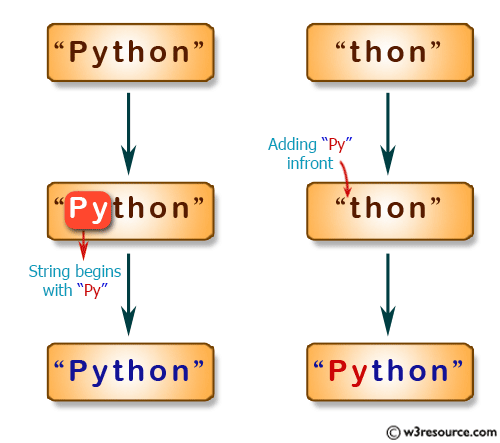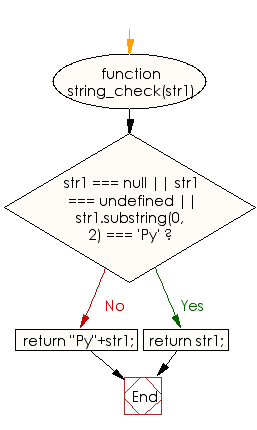# JavaScript: Create a new string adding "Py" in front of a given string

## JavaScript Basic: Exercise-21 with Solution

Write a JavaScript program to create another string by adding "Py" in front of a given string. If the given string begins with "Py" return the original string.

Pictorial Presentation:Sample Solution:

HTML Code:

``````<!DOCTYPE html>
<html>
<meta charset="utf-8">
<meta name="viewport" content="width=device-width">
<title>JavaScript program to create a new string adding “Py” in front of a given string.  If the given string begins with “Py” then return the original string.</title>
<body>

</body>
</html>
```
```

JavaScript Code:

``````function string_check(str1) {
if (str1 === null || str1 === undefined || str1.substring(0, 2) === 'Py')
{
return str1;
}
return "Py"+str1;
}

console.log(string_check("Python"));
console.log(string_check("thon"));
```
```

Sample Output:

```Python
Python
```

Flowchart:ES6 Version:

``````function string_check(str1) {
if (str1 === null || str1 === undefined || str1.substring(0, 2) === 'Py')
{
return str1;
}
return `Py\${str1}`;
}

console.log(string_check("Python"));
console.log(string_check("thon"));
``````

Live Demo:

See the Pen JavaScript: Create new string: basic-ex-21 by w3resource (@w3resource) on CodePen.

Improve this sample solution and post your code through Disqus

What is the difficulty level of this exercise?

Test your Programming skills with w3resource's quiz.

﻿

## JavaScript: Tips of the Day

Example:

```const parseCookie = str =>
str
.split(';')
.map(v => v.split('='))
.reduce((acc, v) => {
acc[decodeURIComponent(v.trim())] = decodeURIComponent(v.trim());
return acc;
}, {});
console.log(parseCookie('foo=bar; equation=E%3Dmc%5E2')); // { foo: 'bar', equation: 'E=mc^2' }
```

Output:

```[object Object] {
equation: "E=mc^2",
foo: "bar"
}
```

We are closing our Disqus commenting system for some maintenanace issues. You may write to us at reach[at]yahoo[dot]com or visit us at Facebook